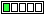All about flooble | fun stuff | Get a free chatterbox | Free JavaScript | Avatarsperplexus dot infoCounting Digit (Posted on 2019-02-25)What is the number of digits of the following product?

11 * 11011 * 1111 * 1010 * 11100111

 No Solution Yet Submitted by Danish Ahmed Khan No RatingComments: ( Back to comment list | You must be logged in to post comments.)how long does this method work? (spoiler) Comment 1 of 1
I'm sure an in-the-head estimate is intended to be the method of solution. That method would be to take the number of digits in one of the five factors given and, for each of the other four factors add one less than the length of that factor.

If we start with the length of the largest factor, 8, we add 1+4+3+3, arriving at a total of 19. Are we right? A calculator gives 1.50862813307147 x 10^18, which indeed has 19 digits if written out.

The estimate was based on the fact that each of the factors was above but "close enough" to some 10^k.

How long would such a method work; that is, how many factors would need to be present to make such an estimate risky?

The estimate is based on the factors being over 10^k but less than (1+1/9)*10^k. The riskiness of course depends on how much less than 1/9 is the amount added to the initial 1, but let's take a worst case, using 1+1/9.

(1+1/9) to what power starts to exceed 10?  What is, what is the base-(1+1/9) logarithm of 10?

log(10)/log(1+1/9) ~= 1/0.04575749056067514
~= 21.85

If we had 22 such factors, we'd start to experience the riskiness.

 Posted by Charlie on 2019-02-25 11:50:29Please log in:

 Search: Search body:
Forums (1)